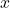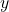# Predictive learning vs. representation learning

When you take a machine learning class, there’s a good chance it’s divided into a unit on supervised learning and a unit on unsupervised learning. We certainly care about this distinction for a practical reason: often there’s orders of magnitude more data available if we don’t need to collect ground-truth labels. But we also tend to think it matters for more fundamental reasons. In particular, the following are some common intuitions:

• In supervised learning, the particular algorithm is usually less important than engineering and tuning it really well. In unsupervised learning, we’d think carefully about the structure of the data and build a model which reflects that structure.
• In supervised learning, except in small-data settings, we throw whatever features we can think of at the problem. In unsupervised learning, we carefully pick the features we think best represent the aspects of the data we care about.
• Supervised learning seems to have many algorithms with strong theoretical guarantees, and unsupervised learning very few.
• Off-the-shelf algorithms perform very well on a wide variety of supervised tasks, but unsupervised learning requires more care and expertise to come up with an appropriate model.

I’d argue that this is deceptive. I think real division in machine learning isn’t between supervised and unsupervised, but what I’ll term predictive learning and representation learning. I haven’t heard it described in precisely this way before, but I think this distinction reflects a lot of our intuitions about how to approach a given machine learning problem.

In predictive learning, we observe data drawn from some distribution, and we are interested in predicting some aspect of this distribution. In textbook supervised learning, for instance, we observe a bunch of pairs, and given some new example, we’re interested in predicting something about the corresponding. In density modeling (a form of unsupervised learning), we observe unlabeled data, and we are interested in modeling the distribution the data comes from, perhaps so we can perform inference in that distribution. In each of these cases, there is a well-defined predictive task where we try to predict some aspect of the observable values possibly given some other aspect.

In representation learning, our goal isn’t to predict observables, but to learn something about the underlying structure. In cognitive science and AI, a representation is a formal system which maps to some domain of interest in systematic ways. A good representation allows us to answer queries about the domain by manipulating that system. In machine learning, representations often take the form of vectors, either real- or binary-valued, and we can manipulate these representations with operations like Euclidean distance and matrix multiplication. For instance, PCA learns representations of data points as vectors. We can ask how similar two data points are by checking the Euclidean distance between them.

In representation learning, the goal isn’t to make predictions about observables, but to learn a representation which would later help us to answer various queries. Sometimes the representations are meant for people, such as when we visualize data as a two-dimensional embedding. Sometimes they’re meant for machines, such as when the binary vector representations learned by deep Boltzmann machines are fed into a supervised classifier. In either case, what’s important is that mathematical operations map to the underlying relationships in the data in systematic ways.

Whether your goal is prediction or representation learning influences the sorts of techniques you’ll use to solve the problem. If you’re doing predictive learning, you’ll probably try to engineer a system which exploits as much information as possible about the data, carefully using a validation set to tune parameters and monitor overfitting. If you’re doing representation learning, there’s no good quantitative criterion, so you’ll more likely build a model based on your intuitions about the domain, and then keep staring at the learned representations to see if they make intuitive sense.

In other words, it parallels the differences I listed above between supervised and unsupervised learning. This shouldn’t be surprising, because the two dimensions are strongly correlated: most supervised learning is predictive learning, and most unsupervised learning is representation learning. So to see which of these dimensions is really the crux of the issue, let’s look at cases where the two differ.

Language modeling is a perfect example of an application which is unsupervised but predictive. The goal is to take a large corpus of unlabeled text (such as Wikipedia) and learn a distribution over English sentences. The problem is motivated by Bayesian models for speech recognition: a distribution over sentences can be used as a prior for what a person is likely to say. The goal, then, is to model the distribution, and any additional structure is unnecessary. Log-linear models, such as that of Mnih et al. , are very good at this, and recurrent neural nets  are even better. These are the sorts of approaches we’d normally apply in a supervised setting: very good at making predictions, but often hard to interpret. One state-of-the-art algorithm for density modeling of text is PAQ , which is a heavily engineered ensemble of sequential predictors, somewhat reminiscent of the winning entries of the Netflix competition.

On the flip side, supervised neural nets are often used to learn representations. One example is Collobert-Weston networks , which attempt to solve a number of supervised NLP tasks by learning representations which are shared between them. Some of the tasks are fairly simple and have a large amount of labeled data, such as predicting which of two words should be used to fill in the blank. Others are harder and have less data available, such as semantic role labeling. The simpler tasks are artificial, and they are there to help learn a representation of words and phrases as vectors, where similar words and phrases map to nearby vectors; this representation should then help performance on the harder tasks. We don’t care about the performance on those tasks per se; we care whether the learned embeddings reflect the underlying structure. To debug and tune the algorithm, we’d focus on whether the representations make intuitive sense, rather than on the quantitative performance. There are no theoretical guarantees that such an approach would work — it all depends on our intuitions of how the different tasks are related.

Based on these two examples, it seems like it’s the predictive/representation dimension which determines how we should approach the problem, rather than supervised/unsupervised.

In machine learning, we tend to think there’s no solid theoretical framework for unsupervised learning. But really, the problem is that we haven’t begun to formally characterize the problem of representation learning. If you just want to build a density modeler, that’s about as well understood as the supervised case. But if the goal is to learn representations which capture the underlying structure, that’s much harder to formalize. In my next post, I’ll try to take a stab at characterizing what representation learning is actually about.

 Mnih, A., and Hinton, G. E. Three new graphical models for statistical language modeling. NIPS 2009

 Sutskever, I., Martens, J., and Hinton, G. E. Generating text with recurrent neural networks. ICML 2011

 Mahoney, M. Adaptive weighting of context models for lossless data compression. Florida Institute of Technology Tech report, 2005

 Collobert, R., and Weston, J. A unified architecture for natural language processing: deep neural networks with multitask learning. ICML 2008